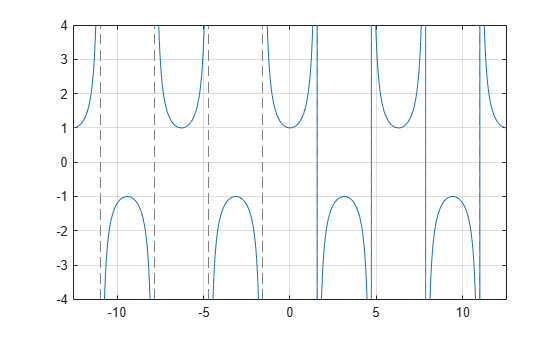Main Content

# sec

Symbolic secant function

## Syntax

``sec(X)``

## Description

example

``sec(X)` returns the secant function of `X`.`

## Examples

### Secant Function for Numeric and Symbolic Arguments

Depending on its arguments, `sec` returns floating-point or exact symbolic results.

Compute the secant function for these numbers. Because these numbers are not symbolic objects, `sec` returns floating-point results.

`A = sec([-2, -pi, pi/6, 5*pi/7, 11])`
```A = -2.4030 -1.0000 1.1547 -1.6039 225.9531```

Compute the secant function for the numbers converted to symbolic objects. For many symbolic (exact) numbers, `sec` returns unresolved symbolic calls.

`symA = sec(sym([-2, -pi, pi/6, 5*pi/7, 11]))`
```symA = [ 1/cos(2), -1, (2*3^(1/2))/3, -1/cos((2*pi)/7), 1/cos(11)]```

Use `vpa` to approximate symbolic results with floating-point numbers:

`vpa(symA)`
```ans = [ -2.4029979617223809897546004014201,... -1.0,... 1.1547005383792515290182975610039,... -1.6038754716096765049444092780298,... 225.95305931402493269037542703557]```

### Plot Secant Function

Plot the secant function on the interval from $-4\pi$ to $4\pi$.

```syms x fplot(sec(x),[-4*pi 4*pi]) grid on```### Handle Expressions Containing Secant Function

Many functions, such as `diff`, `int`, `taylor`, and `rewrite`, can handle expressions containing `sec`.

Find the first and second derivatives of the secant function:

```syms x diff(sec(x), x) diff(sec(x), x, x)```
```ans = sin(x)/cos(x)^2 ans = 1/cos(x) + (2*sin(x)^2)/cos(x)^3```

Find the indefinite integral of the secant function:

`int(sec(x), x)`
```ans = log(1/cos(x)) + log(sin(x) + 1)```

Find the Taylor series expansion of `sec(x)`:

`taylor(sec(x), x)`
```ans = (5*x^4)/24 + x^2/2 + 1```

Rewrite the secant function in terms of the exponential function:

`rewrite(sec(x), 'exp')`
```ans = 1/(exp(-x*1i)/2 + exp(x*1i)/2)```

### Evaluate Units with `sec` Function

`sec` numerically evaluates these units automatically: `radian`, `degree`, `arcmin`, `arcsec`, and `revolution`.

Show this behavior by finding the secant of `x` degrees and `2` radians.

```u = symunit; syms x f = [x*u.degree 2*u.radian]; secf = sec(f)```
```secf = [ 1/cos((pi*x)/180), 1/cos(2)]```

You can calculate `secf` by substituting for `x` using `subs` and then using `double` or `vpa`.

## Input Arguments

collapse all

Input, specified as a symbolic number, variable, expression, or function, or as a vector or matrix of symbolic numbers, variables, expressions, or functions.

## More About

collapse all

### Secant Function

The secant of an angle, α, defined with reference to a right angled triangle isThe secant of a complex argument, α, is

`$\text{sec}\left(\alpha \right)=\frac{2}{{e}^{i\alpha }+{e}^{-i\alpha }}\text{\hspace{0.17em}}.$`

## See Also

Introduced before R2006a

## Support

#### Mathematical Modeling with Symbolic Math Toolbox

Get examples and videos